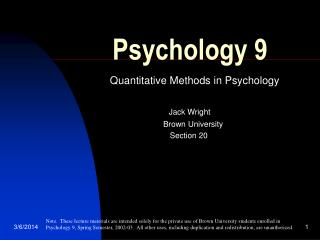# Psychology 9 - PowerPoint PPT PresentationDownload PresentationPsychology 9

Psychology 9
Download Presentation## Psychology 9

- - - - - - - - - - - - - - - - - - - - - - - - - - - E N D - - - - - - - - - - - - - - - - - - - - - - - - - - -
##### Presentation Transcript

1. Psychology 9 Quantitative Methods in Psychology Jack Wright Brown University Section 20 Note. These lecture materials are intended solely for the private use of Brown University students enrolled in Psychology 9, Spring Semester, 2002-03. All other uses, including duplication and redistribution, are unauthorized.

2. Agenda • The t distribution • One sample inference • Two sample inference • Announcements • Bring standard normal and t tables to class • Assignment: Chapter 10 • Exam: next Tuesday • Review: part of Thursday

3. X - m sx Inferences about means WHEN N SMALL… Normally distributed tdf = A variable that Is no longer Normal. Distributed As t, with N - 1 “degrees of freedom” Or df.

4. Using the t distribution • When m is not known, logic of confidence interval using t is the same as before, but we use t instead of z • For confidence intervals using normal: • CIa = X +/- Za* sx • CIa = X +/- Za* sx (with large n) • For confidence intervals using t: • CIa = X +/- tdf,a* sx (with small n) ^ ^

5. Using the t distribution • When m is known, or when some hypothesis about an assumed m is being test, logic of evaluating t is also the same • 1. obtain empirical t: • tobserved = (X - m)/sx • 2. Proceed to evaluate as with z, except that use t distribution with N - 1 df • as before, for “exact” approach: report probability of obtaining observed t: • t(df) = observed t, p < inferred probability. • eg: “t(22) = 3.43, p < .01” • as before, for NHST, “accept” or “reject” null according to pre-established a

6. Illustrative problem using t • A firm claims their prep course increases GRE scores by 8 points, noting that 10 people who took the course had mean = 608, with s=10. Assume all you know is that m for the population = 600. Using a two-sided approach, evaluate the probability of obtaining a result this extreme or more extreme, in either direction.

7. Our t problem • Given: mean = 608; m = 600; s = 10; n = 10. • SEM = s/sqrt(N) = 10/sqrt(10) = 3.16 • Observed t = (608 - 600)/3.16 = 2.53

8. T(9) So, report t(9) = 2.53, p < .04. tobs = 2.53 total area = .016 + .016 = .032. p = ~.016 p = ~.016 -2.53 -2.53

9. Our problem… • As we know, we could also evaluate our result using NHST • set a in advance (e.g., .05, two-tailed) • Locate result in region of acceptance or rejection • “accept” or “reject” null • See next…

10. T(9) tobs = 2.53 acceptance region -2.26 tcrit = 2.26

11. Note on using t tables • See overhead • Walk through

12. Other notes on t • As we know, as n increases, t approaches standard normal • Most t tables stop at df=100 • Switch to normal at that point • However, computers keep computing • So, you will see “t(500) = 2.02, p < .05”, etc. • Key here is to know what you are doing and what this really means

13. Close to actual example of t • “Narragansett--High school principal David G. Andrews, while cautioning that SAT scores are not designed to evaluate teachers, schools, or school systems, has released the latest test results that show Narragansett students’ scores are higher overall than in the previous year…Andrews said the average score climbed 10 points…”

14. Close to actual example of t • Relevant facts: • Mean = 542 • N = 79 students • Assume s = 100 • Infer true mean for last year was 532 • Evaluate this report

15. Close to actual example of t • SEM = s/sqrt(n) = 100/sqrt(79) = 11.25 • t(78) = (mean - m)/SEM • = (542 - 532)/11.25 • = .89 • Df of 60 is closest conservative df to desired df=78 (see table) • So, t critical for 95% CI with df=60 is 2.00 • CI(.95) = 542 +/- 2.00*11.25 • CI(.95) = 542 +/- 22.4 • Note what this means

16. Close to actual example of t • Alternatively, evalute probability of obtaining this result • From table for df=60, probability of |t| = .89 or greater is at least .20, or p > .20 • More exactly, from (much) better table or, more often, from computer, we get: • See next…

17. t(78) So, report: “t(78) = .89, p > .3.” tobs = .89 total area = .188 + .188 = .38 p = ~.188 p = ~.188 -.89 +.89